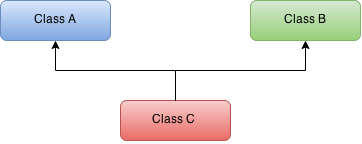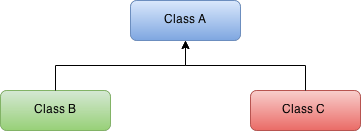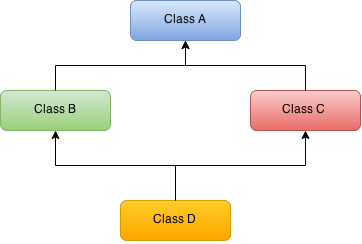## Inheritance in Java :

Inheritance is a key feature of Object-Oriented Programming. It is the method of deriving a new class from an existing class.

Here the main advantage of deriving a class from the existing class is: The derived class acquires all the features of its existing base class.

Hence, inheritance makes a relationship between the two classes: Parent and Child.

The Inheritance relationship means, that the subclass inherits the members of the superclass. Here the members mean instance variables and methods.

For Instance: Benz is a subclass of Car, that means Benz automatically inherits the instance variables and methods of the Car class.

In Java, we can implement the inheritance by using the extends keyword.

## Types Of Inheritance :

Here are the different types of inheritance in Java. Let’s see each type with a neat example.

## Single Inheritance :

This type of inheritance is simple and easy to understand in which one class extends another one class only.In the above diagram, Class A extends Class B, then this type is single inheritance. Here class A is a parent class of class B. That is to say, Class B is a child of Class A.

Example:

SingleInheritance.java
``````class A {
public String meth() {
return "A's Method";
}
}

class B extends A {
public String meth() {
return "B's Method";
}
public String meth2() {
return "B's Method2";
}
}

public class SingleInheritanceDemo {
public static void main(String[] args) {
B b = new B();
System.out.println("B : " + b.meth());
}
}
``````

Output:

``````B : B's Method
``````

## Multiple Inheritance :

Multiple Inheritance means a class is created by deriving from more than one superclass.The above diagram refers to multiple inheritance: Here Class A and Class B are the parent classes. Class C is the child class.

The previous inheritance we learnt had the concept of one base class or parent class. The problem in this type of inheritance is, the derived class will have to manage the dependency on two base classes which are its parents.

This is one of the rarely used inheritance types in software development. Because it leads to some unwanted complexity when further extending the classes. Because of this reason, some of the languages like Java, C# and Smalltalk doesn’t support the Multiple inheritance.

## Multilevel Inheritance :

In this type of inheritance, one class can inherit from a derived class, thereby making this derived class as the base class for the new class.

Why Java does not support Multiple Inheritance?

The reason is to prevent ambiguity. Let us consider a situation in which class C extends class A and Class B and both class A and B have the same method display(). Now java compiler cannot decide, which display method it should inherit. Hence, to prevent such situation, Java does not support multiple inheritance.

This type of inheritance can be depicted as follows:The above diagram represents the multilevel inheritance. Here Class C is extended from Class B and Class B is extended from the Class A.

Example:

MultilevelInheritance.java
``````class A {
public String meth() {
return "A's Method";
}
}

class B extends A {
public String meth() {
return "B's Method";
}
}

class C extends B {
public String meth1() {
return "C's Method";
}
}

public class MultiLevelInheritanceDemo {
public static void main(String[] args) {
A a = new C();
System.out.println("A: " + a.meth());
}
}
``````

Output:

``````A: B's Method
``````

## Hierarchical Inheritance :

In hierarchical inheritance, a single class inherits multiple classes.

##In the above diagram, Class B and Class C are inherited from a single class A. Hence, this represents the Hierarchical inheritance. In other words, A is the parent/base class of Class B and Class C.

Example:

HierarchicalInheritance.java
``````class A {
public String meth() {
return "A's Method";
}
}

class B extends A {
public String meth() {
return "B's Method";
}
}

class C extends A {
public String meth2() {
return "C's Method";
}
}

public class HierarchicallInheritanceDemo {
public static void main(String[] args) {
A a = new C();
System.out.println("C's A : " + a.meth());
B b = new B();
System.out.println("B's B : " + b.meth());
}
}
``````

Output:

``````C's A : A's Method
B's b : B's Method
``````

## Hybrid Inheritance :

The Hybrid inheritance is the combination of both the Single and Multiple inheritance.

We can achieve hybrid inheritance in java in the same way as multiple inheritance by using interfaces. That is, by using interfaces we can have multiple as well as hybrid inheritance in Java.In the above diagram, we can see that Class A extends Class B and Class C and both the classes B and C inherits class D. Thus we can clearly see that this type of inheritance is the combination of single and multiple inheritance.

To sum up, we learnt about the inheritance and its types in this tutorial.

Happy Learning 🙂1.2.